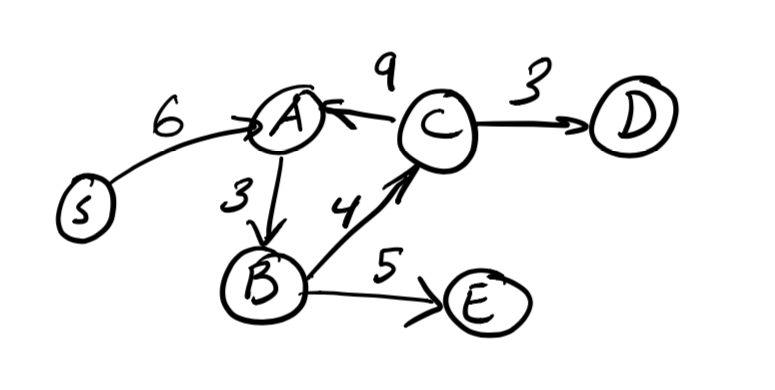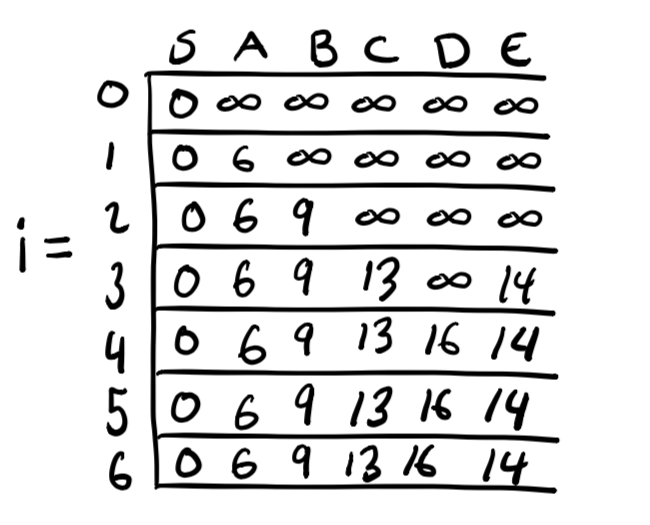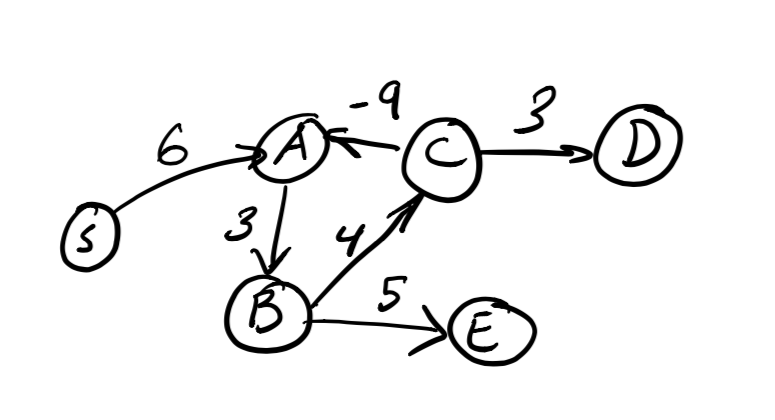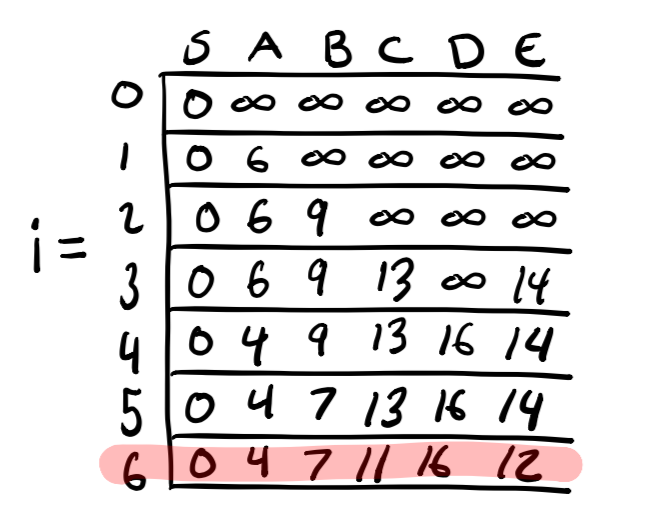Dynamic Programming - Bellman-Ford Algorithm

an exploration of the Bellman-Ford shortest paths algorithm

You may be familiar with Dijkstra’s algorithm for quickly finding the shortest paths in a weighted directed graph. It’s usually great, but if your graph has negative edge weights Dijkstra’s algorithm will let you down. Fortunately we have (slower) alternatives such as the Bellman-Ford and Floyd-Warshall algorithms that do work when we have negative weights. In this post, I’ll talk about how the Bellman-Ford algorithm works to find shortest paths and how we can use it to find negative weight cycles (loops in the graph where the weight ends up decreasing the more times you cycle through the loop).

Shortest Path Between Two Points

Consider the following graph:It has six vertices “S”, “A”, “B”, “C”, “D”, and “E” and six weighted edges between these vertices. We will use the Bellman-Ford algorithm to find the shortest path from our start vertex, “S”, to all of the other reachable vertices in this graph (in this one they’re all reachable from “S”). This is known as the “single-source shortest path problem.”

To explain how Bellman-Ford works using dynamic programming, I’m going to go about solving this problem similarly to my knapsack problem explanation and do the following:

1. State the subproblems
2. Define the recurrence
3. Describe the memoization table structure
4. Show a Python example
5. Perform a quick Big O analysis of the algorithm

Subproblem

The gist of Bellman-Ford is we’re going to consider paths from the start vertex s to some other vertex on the graph z. There are no negative weight cycles on this graph so we only need to visit each vertex at most once. This means our paths can be thought of as traversing up to n edges where n is the number of edges in the graph.

In dynamic programming, we want to find smaller subproblems that we can solve and make use of later. For this problem, we can build off of smaller paths which contain edges of length i where 0 ≤ in - 1.

Let’s define our subproblem as:

D(i, z) = length of the shortest path from s to z using at most i edges

Recurrence

We will first consider the path that has no edges. This basically means we’re stuck at the starting vertex “S” (z = s) and all other vertices are unreachable.

• Base Case: D(0, s) = 0

Now for our main recurrence, we’re basically just going to look at the distance to the vertices that immediately lead to z, we’ll call these y, and choose the smallest one to build off of. Since the number of edges required to get to an earlier vertex y must be shorter than where we’re at now, we know we know that we’ve already solved this distance as part of an earlier subproblem. If these newly reachable paths are smaller than paths to z we found in earlier subproblems we update our shortest path. This recurrence looks like:

• Recurrence: D(i, s) = min(D(i - 1, z), min(D(i - 1, y) + weight(y, z)) for all y with edges leading to z)

Memoization Table Structure

For this example, we’ll use a two-dimensional memoization table to track both i and z. As i increases and we’re able to visit more vertices on the graph, we will track the current shortest path distances at T[i][z]. Vertices that haven’t been reached yet will have shortest path distance of infinity.The table above shows what the memoization table will contain after completing Bellman-Ford for our example graph.

Python Implementation

Below is some Python code demonstrating the core algorithm. It omits some of the data structure setup and helpers, but you can find and run the complete code on Repl.It.

``````graph = {
'S': ['A'],
'A': ['B'],
'B': ['C', 'E'],
'C': ['A', 'D'],
'D': [],
'E': []
}

weights = {
'S': {'A': 6},
'A': {'B': 3},
'B': {'C': 4, 'E': 5},
'C': {'A': -3, 'D': 3},
'D': {},
'E': {}
}

d[vertex_to_idx['S']] = 0

for i in range(1, num_edges):
for z in graph.keys():
z_idx = vertex_to_idx[z]

d = [[math.inf for x in range(num_vertices)] for y in range(num_edges + 1)]

# Initialize the shortest path to z to the
# path found in the previous subproblem.
# Only update if new paths are shorter
d[i][z_idx] = d[i-1][z_idx]

# The reversed graph lets us find which vertexes
for y in reversed_graph[z]:
y_idx = vertex_to_idx[y]
if d[i][z_idx] > (d[i-1][y_idx] + weights[y][z]):
d[i][z_idx] = d[i-1][y_idx] + weights[y][z]
``````

Runnable Code

You can see the code in action below:

Big O Analysis

Referring to the code above, there is some setup which takes linear time (such as reversing the graph), but the bulk of the algorithm’s complexity comes from the three nested loops. To analyze this, let’s have n continue to be the number of edges and let’s introduce m as the number of vertices. The outermost loop is iterating over the n edges and for each loop we then loop over the m vertices. Within this loop, we loop over some subset of the edges leading into z. So effectively we’re doing O(n * m) work here.

This makes the Bellman-Ford algorithm O(nm) where n is the number of edges in the graph and m is the number of vertices.

Finding Negative Weight Cycles Using Bellman-Ford

So our graph above had no negative weight cycles (or negative weights in general for that matter). What happens if we do? Let’s consider the following graph:Notice that there is a negative weight cycle between “A”, “B”, and “C”. Each time we travel around the cycle our total path weight decreases by negative two. The Bellman-Ford algorithm can’t work with this because it means there is no shortest path for certain vertices in the graph – the more times we loop through the cycle the shorter the path gets. This could go on infinitely!

But we can detect negative weight cycles!

Detecting the Cycle

Let’s take a look at a Bellman-Ford memoization table for this graph.If the graph had a well-defined solution we would expect it to converge on the shortest paths after solving for paths of length n - 1 edges (i = 5). As we can see, the paths actually continue to decrease as we check paths of length n (i = 6). This could go on forever, so we can stop the algorithm now. Finding negative weight cycles using Bellman-Ford is as simple as checking to see if the n edge path solution is the same as the n - 1 edge solution. If it’s smaller then there is a negative weight cycle!

One important thing to note, however, is that this will only find cycles for vertices reachable from the start vertex s. If the cycle is not reachable it will not be found by Bellman-Ford. This is a situation where we’d want to turn to the Floyd-Warshall algorithm for finding the shortest paths between all pairs.

That is a post for a future time, though. 🏄‍♂️